Electron. J. Diff. Eqns., Vol. 2008(2008), No. 67, pp. 1-9.

### Impulsive dynamic equations on a time scale Eric R. Kaufmann, Nickolai Kosmatov, Youssef N. Raffoul

Abstract:
Let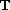be a time scale such that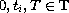,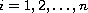, and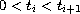. Assume each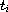is dense. Using a fixed point theorem due to Krasnosel'ski\i}, we show that the impulsive dynamic equation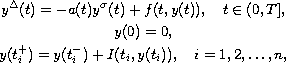where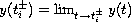, and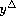is the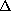-derivative on, has a solution. Under a slightly more stringent inequality we show that the solution is unique using the contraction mapping principle. Finally, with the aid of the contraction mapping principle we study the stability of the zero solution on an unbounded time scale.

Submitted November 20, 2007. Published May 1, 2008.
Math Subject Classifications: 34A37, 34A12, 39A05.
Key Words: Fixed point theory; nonlinear dynamic equation; stability; impulses.

Show me the PDF file (226 KB), TEX file, and other files for this article.Eric R. Kaufmann Department of Mathematics and Statistics University of Arkansas at Little Rock Little Rock, Arkansas 72204-1099, USA email: erkaufmann@ualr.edu Nickolai Kosmatov Department of Mathematics and Statistics University of Arkansas at Little Rock Little Rock, Arkansas 72204-1099, USA email: nxkosmatov@ualr.edu Youssef N. Raffoul Department of Mathematics, University of Dayton Dayton, OH 45469-2316, USA e-mail: youssef.raffoul@notes.udayton.edu